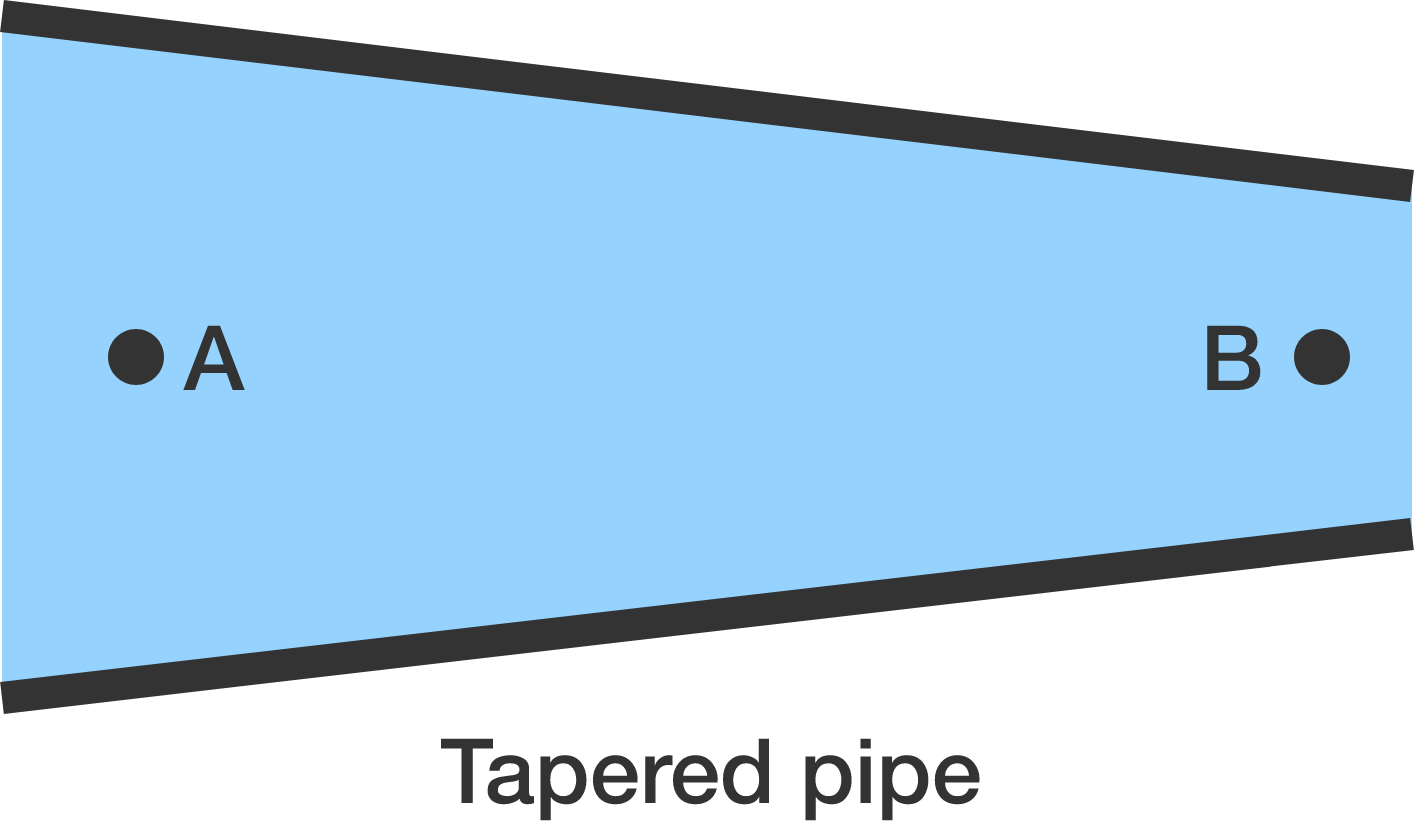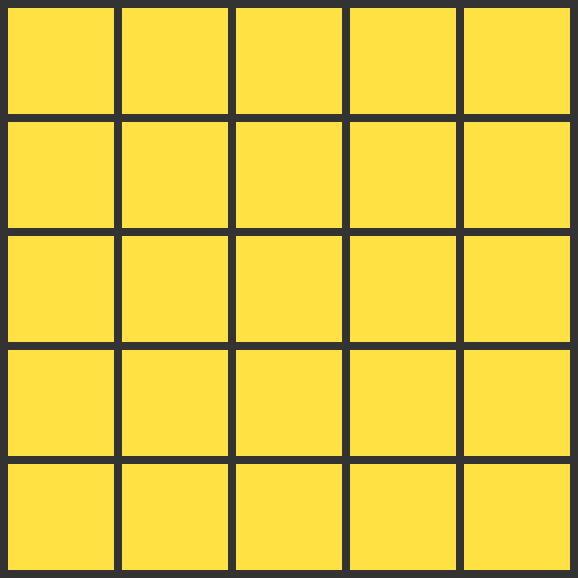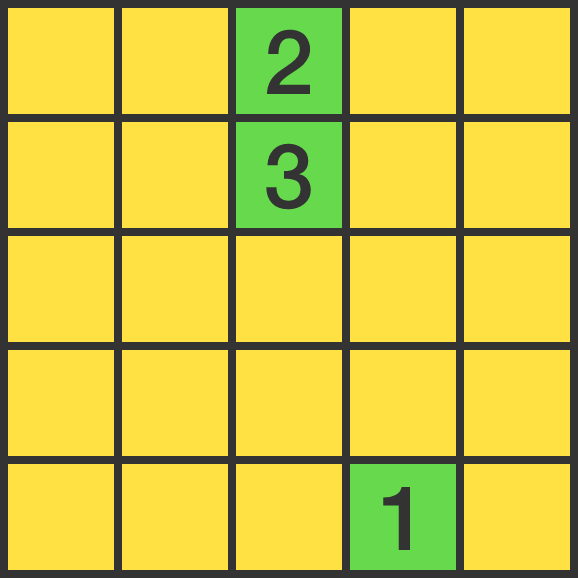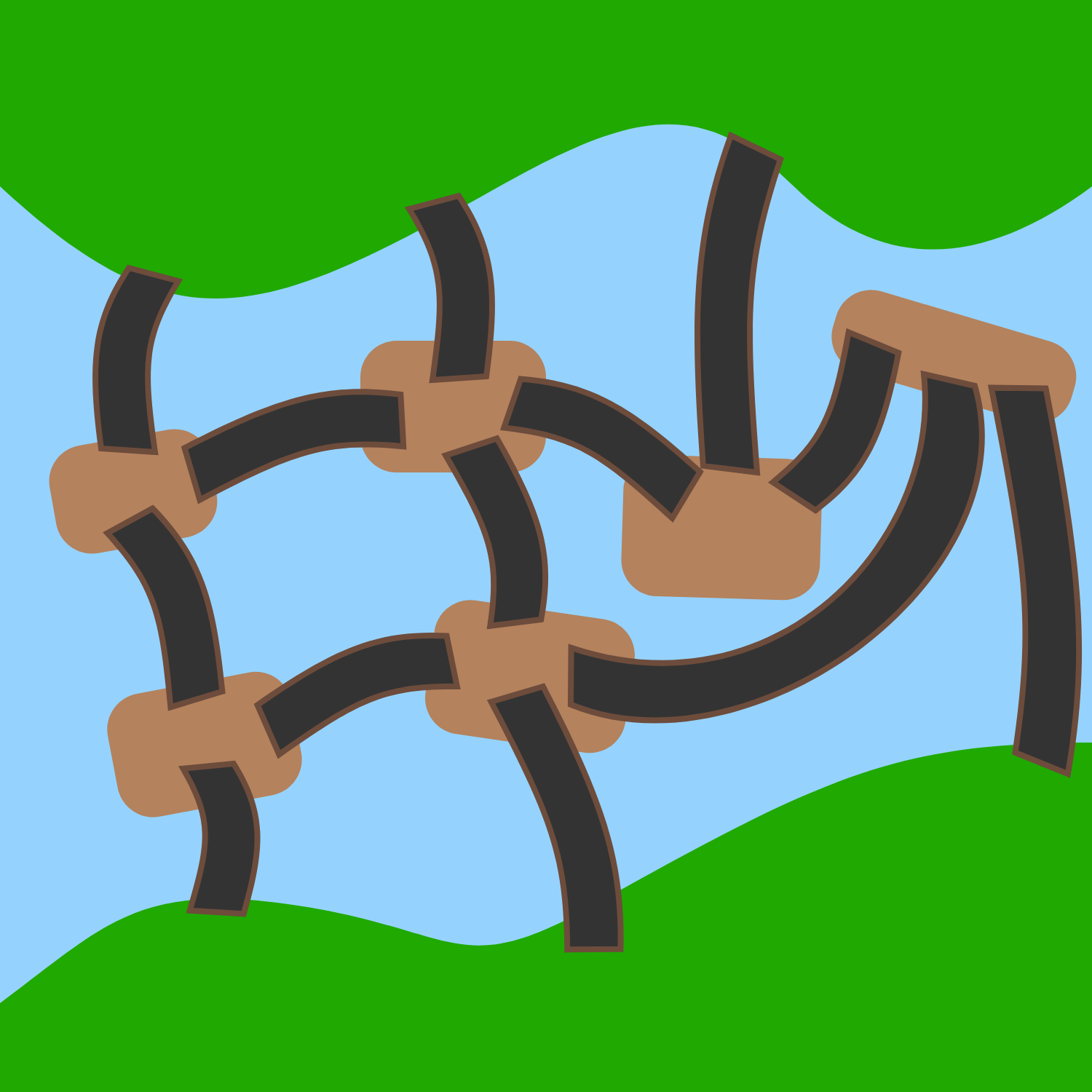# Problems of the Week

Contribute a problem

# 2017-06-19 IntermediateA non-viscous fluid flows steadily in the tapered, horizontal pipe shown above. At which point is the pressure greatest, $\mathbf{A}$ or $\mathbf{B}$?You paint the $5 \times 5$ grid green, one square at a time. You can only paint a square green if it shares an edge with 1 or 3 yellow squares (diagonals don't count).

What's the maximum number of squares you can color?

Below right is an example of a sequence of how you might paint the first 3 squares.Clarification: The square colored green with sequence number 2 now borders two yellow squares, but it was bordering three yellow squares at the time of coloring.

$\large \sqrt{2+\sqrt{5}}+\sqrt{2-\sqrt{5}}$

My calculator says that the above expression equals 1.

But is the answer correct? Or, is 1 just an approximation?

Fill in the blank:

$N$ has precisely 10 positive divisors.
$2N$ has precisely 15 positive divisors.
$3N$ has precisely 20 positive divisors.
$4N$ has precisely $\text{\_\_\_\_\_\_\_\_}$ positive divisors.In the image on the right, there is a river running between 2 banks (marked in green). There are 6 islands (marked in brown) in the river, and these land masses are connected by 13 bridges (marked in black). The inhabitants can only use these bridges to get across the river (no swimming, boats, etc. are allowed).

One day, an extremely heavy storm destroys at least one of the bridges, which prevents the inhabitants from crossing directly between the connected land masses. Out of the $2^{13} - 1 = 8191$ cases where at least one bridge is destroyed, in how many cases can the inhabitants still cross between the two banks?

×